Home Practice
For learners and parents For teachers and schools
Textbooks
Full catalogue
Pricing SupportLog in

We think you are located in United States. Is this correct?

# 6.5 Exponential functions

## 6.5 Exponential functions (EMA4V)

### Functions of the form $$y={b}^{x}$$ (EMA4W)

Functions of the general form $$y=a{b}^{x}+q$$ are called exponential functions. In the equation $$a$$ and $$q$$ are constants and have different effects on the function.

## Worked example 12: Plotting an exponential function

$$y=f(x)={b}^{x} \text{ for } b>0 \text{ and } b\ne 1$$

Complete the following table for each of the functions and draw the graphs on the same system of axes: $$f(x)={2}^{x}$$, $$g(x)={3}^{x}$$, $$h(x)={5}^{x}$$.

 $$-\text{2}$$ $$-\text{1}$$ $$\text{0}$$ $$\text{1}$$ $$\text{2}$$ $$f(x)={2}^{x}$$ $$g(x)={3}^{x}$$ $$h(x)={5}^{x}$$
1. At what point do these graphs intersect?

2. Explain why they do not cut the $$x$$-axis.

3. Give the domain and range of $$h(x)$$.

4. As $$x$$ increases, does $$h(x)$$ increase or decrease?

5. Which of these graphs increases at the slowest rate?

6. For $$y={k}^{x}$$ and $$k>1$$, the greater the value of $$k$$, the steeper the curve of the graph. True or false?

Complete the following table for each of the functions and draw the graphs on the same system of axes: $$F(x)={\left(\dfrac{1}{2}\right)}^{x}$$, $$G(x)={\left(\dfrac{1}{3}\right)}^{x}$$, $$H(x)={\left(\dfrac{1}{5}\right)}^{x}$$

 $$-\text{2}$$ $$-\text{1}$$ $$\text{0}$$ $$\text{1}$$ $$\text{2}$$ $$F(x)={\left(\frac{1}{2}\right)}^{x}$$ $$G(x)={\left(\frac{1}{3}\right)}^{x}$$ $$H(x)={\left(\frac{1}{5}\right)}^{x}$$
1. Give the $$y$$-intercept for each function.

2. Describe the relationship between the graphs $$f(x)$$ and $$F(x)$$.

3. Describe the relationship between the graphs $$g(x)$$ and $$G(x)$$.

4. Give the domain and range of $$H(x)$$.

5. For $$y={\left(\dfrac{1}{k}\right)}^{x}$$ and $$k>1$$, the greater the value of $$k$$, the steeper the curve of the graph. True or false?

6. Give the equation of the asymptote for the functions.

### Substitute values into the equations

 $$-\text{2}$$ $$-\text{1}$$ $$\text{0}$$ $$\text{1}$$ $$\text{2}$$ $$f(x)={2}^{x}$$ $$\frac{1}{4}$$ $$\frac{1}{2}$$ $$\text{1}$$ $$\text{2}$$ $$\text{4}$$ $$g(x)={3}^{x}$$ $$\frac{1}{9}$$ $$\frac{1}{3}$$ $$\text{1}$$ $$\text{3}$$ $$\text{9}$$ $$h(x)={5}^{x}$$ $$\frac{1}{25}$$ $$\frac{1}{5}$$ $$\text{1}$$ $$\text{5}$$ $$\text{25}$$
 $$-\text{2}$$ $$-\text{1}$$ $$\text{0}$$ $$\text{1}$$ $$\text{2}$$ $$F(x)={\left(\frac{1}{2}\right)}^{x}$$ $$\text{4}$$ $$\text{2}$$ $$\text{1}$$ $$\frac{1}{2}$$ $$\frac{1}{4}$$ $$G(x)={\left(\frac{1}{3}\right)}^{x}$$ $$\text{9}$$ $$\text{3}$$ $$\text{1}$$ $$\frac{1}{3}$$ $$\frac{1}{9}$$ $$H(x)={\left(\frac{1}{5}\right)}^{x}$$ $$\text{25}$$ $$\text{5}$$ $$\text{1}$$ $$\frac{1}{5}$$ $$\frac{1}{25}$$

### Plot the points and join with a smooth curve

1. We notice that all graphs pass through the point $$(0;1)$$. Any number with exponent $$\text{0}$$ is equal to $$\text{1}$$.

2. The graphs do not cut the $$x$$-axis because you can never get $$\text{0}$$ by raising any non-zero number to the power of any other number.

3. Domain: $$\left\{x:x\in \mathbb{R}\right\}$$

Range: $$\left\{y:y\in \mathbb{R}, y>0\right\}$$

4. As $$x$$ increases, $$h(x)$$ increases.

5. $$f(x)={2}^{x}$$ increases at the slowest rate because it has the smallest base.

6. True: the greater the value of $$k$$ $$\left(k>1\right)$$, the steeper the graph of $$y={k}^{x}$$.

1. The $$y$$-intercept is the point $$(0;1)$$ for all graphs. For any real number $$z$$: $${z}^{0}=1 \qquad z \ne 0$$.

2. $$F(x)$$ is the reflection of $$f(x)$$ about the $$y$$-axis.

3. $$G(x)$$ is the reflection of $$g(x)$$ about the $$y$$-axis.

4. Domain: $$\left\{x:x\in \mathbb{R}\right\}$$

Range: $$\left\{y:y\in \mathbb{R}, y>0\right\}$$

5. True: the greater the value of $$k$$ $$\left(k>1\right)$$, the steeper the graph of $$y={\left(\frac{1}{k}\right)}^{x}$$.

6. The equation of the horizontal asymptote is $$y=0$$, the $$x$$-axis.

### Functions of the form $$y=a{b}^{x}+q$$ (EMA4X)

CAPS states to only investigate the effects of $$a$$ and $$q$$ on an exponential graph. However it is also important for learners to see that $$b$$ has a different effect on the graph depending on if $$b > 1$$ or $$0 < b < 1$$.

For this reason the effect of $$b$$ is included in the investigation so that learners can see what happens when $$b > 1$$ and when $$0 < b < 1$$.

Also note that the above worked example further reinforces the effects of $$b$$ on the exponential graph.

## The effects of $$a$$, $$q$$ and $$b$$ on an exponential graph.

On the same set of axes, plot the following graphs ($$a=1$$, $$q=0$$ and $$b$$ changes):

1. $$y_{1} = 2^{x}$$

2. $$y_{2} = \left(\frac{1}{2}\right)^{x}$$

3. $$y_{3} = 6^{x}$$

4. $$y_{4} = \left(\frac{1}{6}\right)^{x}$$

 $$-\text{2}$$ $$-\text{1}$$ $$\text{0}$$ $$\text{1}$$ $$\text{2}$$ $$y_{1}=2^{x}$$ $$y_{2}=\left(\frac{1}{2}\right)^{x}$$ $$y_{3}=6^{x}$$ $$y_{4}=\left(\frac{1}{6}\right)^{x}$$

Use your results to deduce the effect of $$b$$.

On the same set of axes, plot the following graphs ($$b=2$$, $$a=1$$ and $$q$$ changes):

1. $${y}_{5}={2}^{x}-2$$

2. $${y}_{6}={2}^{x}-1$$

3. $${y}_{7}={2}^{x}$$

4. $${y}_{8}={2}^{x}+1$$

5. $${y}_{9}={2}^{x}+2$$

 $$-\text{2}$$ $$-\text{1}$$ $$\text{0}$$ $$\text{1}$$ $$\text{2}$$ $${y}_{5}={2}^{x}-2$$ $${y}_{6}={2}^{x}-1$$ $${y}_{7}={2}^{x}$$ $${y}_{8}={2}^{x}+1$$ $${y}_{9}={2}^{x}+2$$

Use your results to deduce the effect of $$q$$.

On the same set of axes, plot the following graphs ($$b=2$$, $$q=0$$ and $$a$$ changes).

1. $${y}_{10}=1 \times {2}^{x}$$

2. $${y}_{11}=2\times {2}^{x}$$

3. $${y}_{12}=-1 \times {2}^{x}$$

4. $${y}_{13}=-2\times {2}^{x}$$

 $$-\text{2}$$ $$-\text{1}$$ $$\text{0}$$ $$\text{1}$$ $$\text{2}$$ $${y}_{10}=1 \times {2}^{x}$$ $${y}_{11}=2\times {2}^{x}$$ $${y}_{12}=-1 \times {2}^{x}$$ $${y}_{13}=-2\times {2}^{x}$$

Use your results to deduce the effect of $$a$$.

The effect of $$q$$

The effect of $$q$$ is called a vertical shift because all points are moved the same distance in the same direction (it slides the entire graph up or down).

• For $$q>0$$, the graph is shifted vertically upwards by $$q$$ units.

• For $$q<0$$, the graph is shifted vertically downwards by $$q$$ units.

The horizontal asymptote is shifted by $$q$$ units and is the line $$y=q$$.

The effect of $$a$$

The sign of $$a$$ determines whether the graph curves upwards or downwards.

For $$0 < b < 1$$:

• For $$a>0$$, the graph curves downwards. It reflects the graph about the horizontal asymptote.

• For $$a<0$$, the graph curves upwards.

For $$b > 1$$:

• For $$a>0$$, the graph curves upwards.

• For $$a<0$$, the graph curves downwards. It reflects the graph about the horizontal asymptote.

 $$b>1$$ $$a<0$$ $$a>0$$ $$q>0$$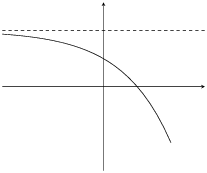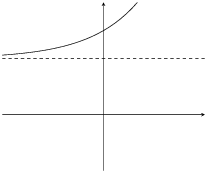$$q<0$$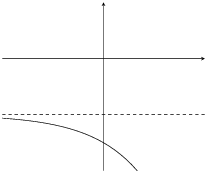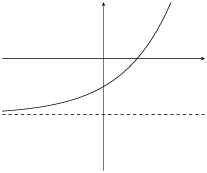The effect of $$a$$ and $$q$$ on an exponential graph when $$b > 1$$.

 $$00$$ $$q>0$$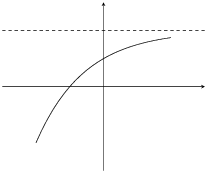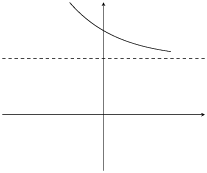$$q<0$$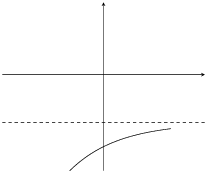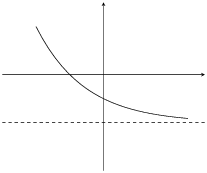The effect of $$a$$ and $$q$$ on an exponential graph when $$0 < b < 1$$.

### Discovering the characteristics (EMA4Y)

The standard form of an exponential function is $$y=a{b}^{x}+q$$.

#### Domain and range

For $$y=a{b}^{x}+q$$, the function is defined for all real values of $$x$$. Therefore the domain is $$\left\{x:x\in \mathbb{R}\right\}$$.

The range of $$y=a{b}^{x}+q$$ is dependent on the sign of $$a$$.

For $$a>0$$:

\begin{align*} {b}^{x}& > 0 \\ a{b}^{x}& > 0 \\ a{b}^{x}+q& > q \\ f(x)& > q \end{align*}

Therefore, for $$a>0$$ the range is $$\left\{f(x):f(x)>q\right\}$$.

For $$a<0$$:

\begin{align*} {b}^{x}& > 0 \\ a{b}^{x}& < 0 \\ a{b}^{x}+q& < q \\ f(x)& < q \end{align*}

Therefore, for $$a<0$$ the range is $$\left\{f(x):f(x)<q\right\}$$.

## Worked example 13: Domain and range of an exponential function

Find the domain and range of $$g(x)=5 \cdot {2}^{x}+1$$

### Find the domain

The domain of $$g(x)=5\times {2}^{x}+1$$ is $$\left\{x:x\in \mathbb{R}\right\}$$.

### Find the range

\begin{align*} {2}^{x}& > 0 \\ 5\times {2}^{x}& > 0 \\ 5\times {2}^{x}+1& > 1 \end{align*}

Therefore the range is $$\left\{g(x):g(x)>1\right\}$$.

#### Intercepts

The $$y$$-intercept.

For the $$y$$-intercept, let $$x=0$$:

\begin{align*} y& = a{b}^{x}+q \\ & = a{b}^{0}+q \\ & = a(1)+q \\ & = a+q \end{align*}

For example, the $$y$$-intercept of $$g(x)=5\times {2}^{x}+1$$ is given by setting $$x=0$$:

\begin{align*} y& = 5\times {2}^{x}+1 \\ & = 5\times {2}^{0}+1 \\ & = 5+1 \\ & = 6 \end{align*}

This gives the point $$(0;6)$$.

The $$x$$-intercept:

For the $$x$$-intercept, let $$y=0$$.

For example, the $$x$$-intercept of $$g(x)=5\times {2}^{x}+1$$ is given by setting $$y=0$$:

\begin{align*} y& = 5\times {2}^{x}+1 \\ 0& = 5\times {2}^{x}+1 \\ -1& = 5\times {2}^{x} \\ {2}^{x}& = -\frac{1}{5} \end{align*}

There is no real solution. Therefore, the graph of $$g(x)$$ does not have any $$x$$-intercepts.

#### Asymptotes

Exponential functions of the form $$y=a{b}^{x}+q$$ have a single horizontal asymptote, the line $$x=q$$.

### Sketching graphs of the form $$y=a{b}^{x}+q$$ (EMA4Z)

In order to sketch graphs of functions of the form, $$y=a{b}^{x}+q$$, we need to determine four characteristics:

1. sign of $$a$$

2. $$y$$-intercept

3. $$x$$-intercept

4. asymptote

The following video shows some examples of sketching exponential functions.

Video: 2FYW

## Worked example 14: Sketching an exponential function

Sketch the graph of $$g(x)=3\times {2}^{x}+2$$. Mark the intercept and the asymptote.

### Examine the standard form of the equation

From the equation we see that $$a>1$$, therefore the graph curves upwards. $$q>0$$ therefore the graph is shifted vertically upwards by $$\text{2}$$ units.

### Calculate the intercepts

For the $$y$$-intercept, let $$x=0$$:

\begin{align*} y& = 3\times {2}^{x}+2 \\ & = 3\times {2}^{0}+2 \\ & = 3+2 \\ & = 5 \end{align*}

This gives the point $$(0;5)$$.

For the $$x$$-intercept, let $$y=0$$:

\begin{align*} y& = 3\times {2}^{x}+2 \\ 0& = 3\times {2}^{x}+2 \\ -2& = 3\times {2}^{x} \\ {2}^{x}& = -\frac{2}{3} \end{align*}

There is no real solution, therefore there is no $$x$$-intercept.

### Determine the asymptote

The horizontal asymptote is the line $$y=2$$.

### Plot the points and sketch the graph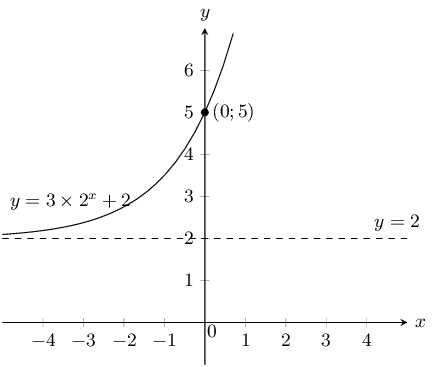Domain: $$\left\{x:x\in \mathbb{R}\right\}$$

Range: $$\left\{g(x):g(x)>2\right\}$$

Note that there is no axis of symmetry for exponential functions.

## Worked example 15: Sketching an exponential graph

Sketch the graph of $$y=-2\times {3}^{x}+6$$

### Examine the standard form of the equation

From the equation we see that $$a<0$$ therefore the graph curves downwards. $$q>0$$ therefore the graph is shifted vertically upwards by $$\text{6}$$ units.

### Calculate the intercepts

For the $$y$$-intercept, let $$x=0$$:

\begin{align*} y& = -2\times {3}^{x}+6 \\ & = -2\times {3}^{0}+6 \\ & = 4 \end{align*}

This gives the point $$(0;4)$$.

For the $$x$$-intercept, let $$y=0$$:

\begin{align*} y& = -2\times {3}^{x}+6 \\ 0& = -2\times {3}^{x}+6 \\ -6& = -2\times {3}^{x} \\ {3}^{1}& = {3}^{x} \\ \therefore x& = 1 \end{align*}

This gives the point $$(1;0)$$.

### Determine the asymptote

The horizontal asymptote is the line $$y=6$$.

### Plot the points and sketch the graph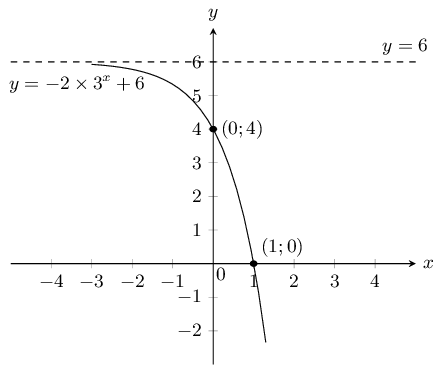Domain: $$\left\{x:x\in \mathbb{R}\right\}$$

Range: $$\left\{g(x):g(x)<6\right\}$$

Textbook Exercise 6.5

Given the following equation:

$$y = -\frac{2}{3}. (\text{3})^{x} + 1$$

Calculate the $$y$$-intercept. Your answer must be correct to 2 decimal places.

\begin{align*} y & = \left( -\frac{2}{3} \right). (\text{3})^{x} + \text{1} \\ & = \left( -\frac{2}{3} \right). (\text{3})^{(0)} + \text{1} \\ & = \left( -\frac{2}{3} \right). (\text{1}) + \text{1} \\ & = (-\text{0,66666...}) + \text{1} \\ & = \text{0,33} \end{align*}

The $$y$$-intercept is $$(0;\text{0,33})$$.

Now calculate the $$x$$-intercept. Estimate your answer to one decimal place if necessary.

We calculate the $$x$$-intercept by letting $$y = 0$$. Then we solve for $$x$$:

\begin{align*} 0 & = \left( -\frac{2}{3} \right). (\text{3})^{x} + \text{1} \\ -\text{1} & = \left( -\frac{2}{3} \right)(\text{3})^{x} \\ \left( - \frac{3}{2} \right) (-\text{1}) & = \left( - \frac{3}{2} \right) \left( - \frac{2}{3} \right). (\text{3})^{x} \\ \frac{3}{2} & = \text{3}^{x} \end{align*}

To find the answer we try different values of $$x$$:

\begin{align*} \text{Try: } \text{3}^{-1} & = \text{0,333...}\\ \text{Try: } \text{3}^0 & = \text{1}\\ \text{Try: } \text{3}^1 & = \text{3} \end{align*}

We can see that the exponent must be between 0 and 1. Next we try values starting with $$\text{0,1}$$ and see what the value of the exponent is. Doing this we find that $$x = \text{0,4}$$.

The $$x$$-intercept is $$(\text{0,4};0)$$.

The graph here shows an exponential function with the equation $$y = a \cdot 2^{x} + q$$. One point is given on the curve: Point A is at $$(-3;\text{3,875})$$. Determine the values of $$a$$ and $$q$$, correct to the nearest integer.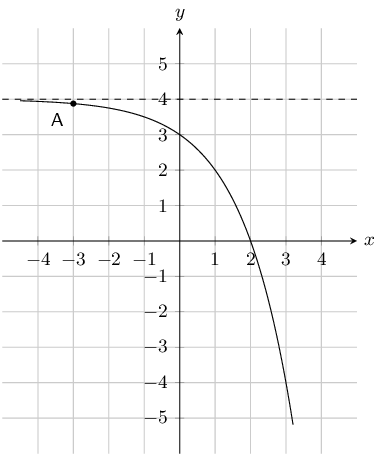The asymptote lies at $$y = 4$$. Therefore $$q$$ is 4.

At this point we know that the equation for the graph must be $$y = a \cdot 2^{x} + 4$$.

\begin{align*} y & = a (2)^{x} + 4 \\ (\text{3,875}) & = a (2)^{(-3)} + 4 \\ \text{3,875} - 4 & = a (2)^{\left(-3\right)} \\ -\text{0,125} & = a (\text{0,125}) \\ -1 & = a \end{align*}

Therefore $$a = -1 \text{ and } q = 4$$

Below you see a graph of an exponential function with the equation $$y = a \cdot 2^{x} + q$$. One point is given on the curve: Point A is at $$(-3;\text{4,875})$$. Calculate the values of $$a$$ and $$q$$, correct to the nearest integer.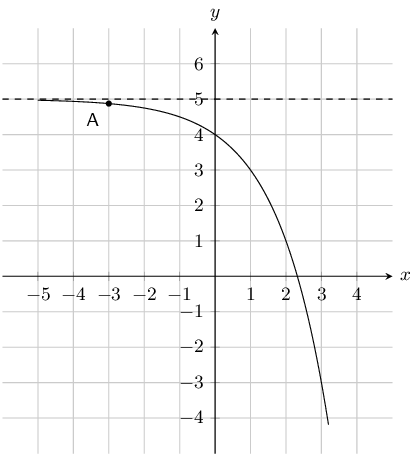The asymptote lies at $$y = 5$$. Therefore $$q$$ is 5.

At this point we know that the equation for the graph must be $$y = a \cdot 2^{x} + 5$$.

\begin{align*} y & = a (2)^{x} + 5 \\ (\text{4,875}) & = a (2)^{ (-3)} + 5 \\ \text{4,875} - 5 & = a (2)^{\left(-3\right)} \\ -\text{0,125} & = a (\text{0,125}) \\ -1 & = a \end{align*}

Therefore $$a = -1 \text{ and } q = 5$$.

Given the following equation:

$$y = \dfrac{1}{4} \cdot (4)^{x} - 1$$

Calculate the $$y$$-intercept. Your answer must be correct to 2 decimal places.

\begin{align*} y & = \left( \frac{1}{4} \right)\cdot(4)^{x} - 1 \\ & = \left( \frac{1}{4} \right) \cdot (4)^{(0)} -\text{1} \\ & = \left( \frac{1}{4} \right) \cdot (1) - 1 \\ & = (\text{0,25}) - 1 \\ & = -\text{0,75} \end{align*}

Therefore the $$y$$-intercept is $$(0;-\text{0,75})$$.

Now calculate the $$x$$-intercept.

We calculate the $$x$$-intercept by letting $$y = 0$$. Then start to solve for $$x$$.

\begin{align*} 0 & = \left( \frac{1}{4} \right) \cdot (4)^{x} - 1 \\ 1 & = \left( \frac{1}{4} \right) \cdot (4)^{x} \\ \left( 4 \right) (1) & = \left( 4 \right) \left( \frac{1}{4} \right) \cdot (4)^{x} \\ 4^1 & = 4^{x} \\ x &= 1 \end{align*}

Therefore the $$x$$-intercept is $$(1;0)$$.

Given the following graph, identify a function that matches each of the following equations: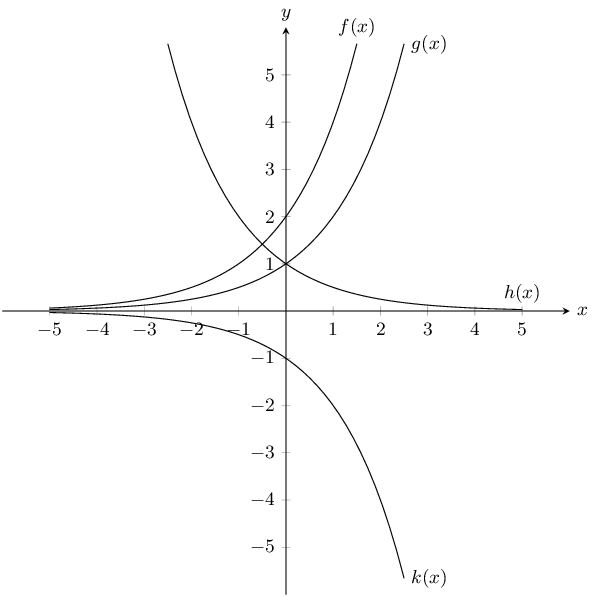$$y = 2^x$$

$$g(x)$$

$$y = -2^x$$

$$k(x)$$

$$y = 2 \cdot 2^x$$

$$f(x)$$

$$y = \left(\frac{1}{2}\right)^{x}$$

$$h(x)$$

Given the functions $$y=2^{x}$$ and $$y=\left(\frac{1}{2}\right)^{x}$$.

Draw the graphs on the same set of axes.

For $$y=2^{x}$$:

$$a$$ is positive and greater than 1 and so the graph curves upwards. The $$y$$-intercept is $$(0;1)$$. There is no $$x$$-intercept. The asymptote is the line $$x = 0$$.

For $$y=\left(\frac{1}{2}\right)^{x}$$:

$$a$$ is positive and less than 1 and so the graph curves downwards. The $$y$$-intercept is $$(0;1)$$. There is no $$x$$-intercept. The asymptote is the line $$x = 0$$.

The graph is: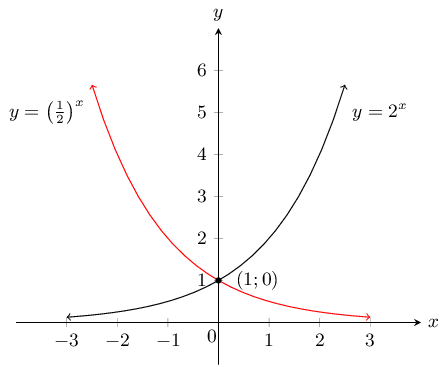Is the $$x$$-axis an asymptote or an axis of symmetry to both graphs? Explain your answer.

The $$x$$-axis is an asymptote to both graphs because both approach the $$x$$-axis but never touch it.

Which graph can be described by the equation $$y=2^{-x}$$? Explain your answer.

$$y= \left(\frac{1}{2}\right)^{x}$$ can be described by the equation $$y=2^{-x}$$ because $$y=\left(\frac{1}{2}\right)^x = \left(2^{-1} \right)^x = 2^{-x}$$.

Solve the equation $$2^{x} = \left(\frac{1}{2}\right)^{x}$$ graphically and check your answer is correct by using substitution.

The graphs intersect at the point $$(0;1)$$. If we substitute these values into each side of the equation we get:

\begin{align*} \text{LHS: } 2^{x} & = 2^0 = 1 \text{ and} \\ \text{RHS: } (\frac{1}{2})^x & = (\frac{1}{2})^0 = 1 \end{align*}

LHS = RHS, therefore the answer is correct.

The curve of the exponential function $$f$$ in the accompanying diagram cuts the $$y$$-axis at the point $$A(0;1)$$ and passes through the point $$B(2;9)$$.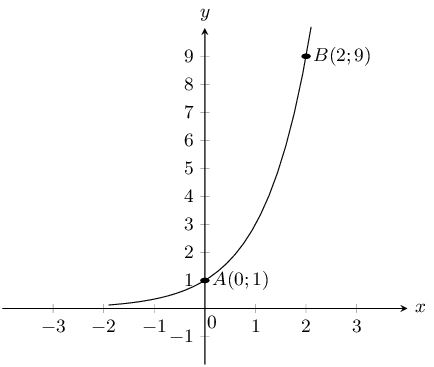Determine the equation of the function $$f$$.

The general form of the equation is $$f(x)=a \cdot b^x+q$$.

We are given $$A(0; 1)$$ and $$B(2; 9)$$.

The asymptote is at $$y = 0$$ and so $$q = 0$$.

Substitute in the values of point $$A$$:

\begin{align*} 1 & = a \cdot b^0 \\ 1 & = a \end{align*}

Substitute in the values of point $$B$$:

\begin{align*} 9 & = b^2 \\ 3^2 & = b^2\\ \therefore b & = 3 \end{align*}

Therefore the equation is $$f(x) = 3^x$$.

Determine the equation of the function $$h(x)$$, the reflection of $$f(x)$$ in the $$x$$-axis.

$$h(x)=-3^{x}$$

Determine the range of $$h(x)$$.

Range: $$(-\infty;0)$$

Determine the equation of the function $$g(x)$$, the reflection of $$f(x)$$ in the $$y$$-axis.

$$g(x)=3^{-x}$$

Determine the equation of the function $$j(x)$$ if $$j(x)$$ is a vertical stretch of $$f(x)$$ by $$+2$$ units.

$$j(x)=2 \cdot 3^{x}$$

Determine the equation of the function $$k(x)$$ if $$k(x)$$ is a vertical shift of $$f(x)$$ by $$-3$$ units.

$$k(x)=3^{x}-3$$